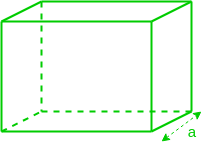Open in App
Not now

# Cubes

• Difficulty Level : Medium
• Last Updated : 09 Jun, 2022

A type of cuboid in which all the sides i.e length, breadth & height are equal. All faces of cubes are of the same area.• In a cube, there are 8-corners/vertices
• There are 6-faces(all equal in area)
• There are 12 edges(all equal in length)
`No. of edges = (No. of vertices) + (No. of faces) - 2`

Primarily question in cubes are based on the painting of faces of cubes & then cutting the painted cube into identical cubelets. Because question are based on the way cube is painted & how cuts are being made, hence we don’t recommend any thumb rule. Still however because some basic rules are always applicable which we will be using as building blocks for answering the question.

• If n equidistant cuts are made(all parallel to the same surface), the cube will be divided into (n + 1) identical cuboidal pieces with each such cut there will 2a2 new surface area will be generated which will be unpainted.
• If we want to cut our bigger cube into identical n3 cubelets, using minimum number cuts, we need total 3(n – 1) cuts, such that (n – 1) cuts parallel to each of these faces which are joining to corner.
• If the number of cuts is not multiple of three then cube can never be cut into identical cubes but still it can be cut into the maximum number of identical cuboidal pieces. To maximize such number of pieces we need to split the number of cuts into three parts which are closest.

Directions for Example (1-4): If a cube is cut into n3 identical cubelets using minimum no. of cuts, after painting all faces of the cube with white color, then answer the following questions.

Example-1: What is the maximum number of possible painted faces in once of such of cubelets?

Solution – Maximum faces painted will be three in any such cubelets, which will be in case of cubelets coming out of corners of big cube after cutting.

Example-2: What is the minimum number of cuts required?

Solution – Total number cuts required in 3(n – 1). (n – 1) equidistant cuts parallel to each of 3 faces which are joining to corner.

Example-3: How many cubelets will have at most 2 faces painted?

Solution – To find out the number of cubelets with at most 2 faces painted, we need to remove all those cubes which have exactly 3 faces painted = Total no. of cubes – No. of cubes with 3 faces painted = (n3 – 8)

Example-4: How many cubelets will have at least 1 face painted?

Solution – To find out the number of cubelets with at least one face painted, we need to remove all the cubelets which have no face painted = n3 – (n – 2)3

Directions for Example (5-6): A cube is divided into 343 identical cubelets.Each cut is made parallel to some surface of the cube. But before doing that the cube is colored with green color on one set of adjacent faces, red on the second and blue on the third set.

Example-5: How many minimum cuts you have made? (a)15 (b)18 (c)21 (d)9

Solution –

```n3 = 343 = 73
==> n = 7 ```

Minimum number of cuts = 3(n -1)

```= 3(7 - 1)
= 3 X 6
= 18```

Example-6: How many cubelets are coloured with exactly two colours? (a)59 (b)63 (c)51 (d)54

Solution –

```n3 = 343 = 73
==> n = 7 ```

Number of cubelet with no face painted will

```= (n - 2)3
= (7 - 2)3
= 125 ```

No. of cubelet with 2 color = Total no. of cubelet – [cubelets with one color + cubelets with no color + cubelets with three color]

```= 343 - [125 + 2 + 165]
= 51```
My Personal Notes arrow_drop_up
Related Articles